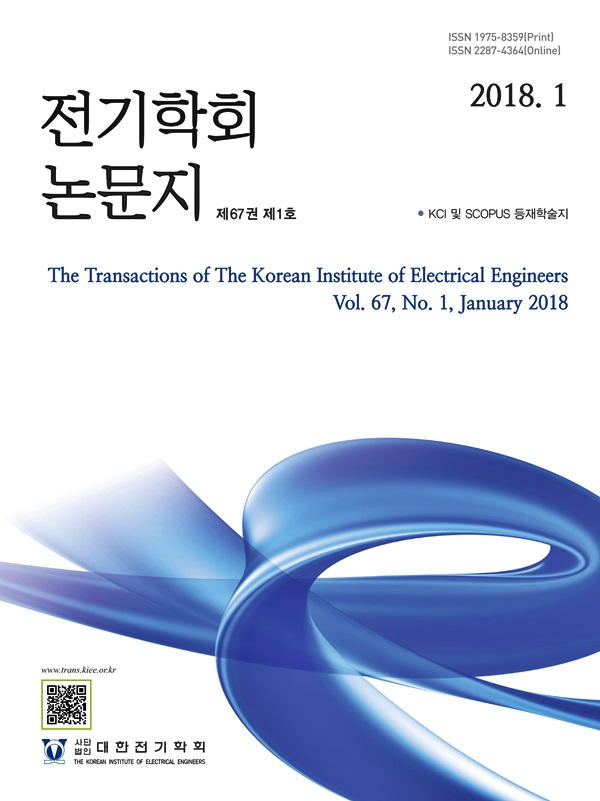Mobile QR Code#### The Transactions of the Korean Institute of Electrical Engineers

##### ISO Journal TitleTrans. Korean. Inst. Elect. Eng.
• SCOPUS
• KCI Accredited Journal
Title Probabilistic Electricity Load Forecasting Algorithm Based on the Gradient Boosting Machine and Laplace Distribution 박세준(Sejun Park) ; 김현진(Hyunjin Kim) ; 이두희(Duehee Lee) https://doi.org/10.5370/KIEE.2021.70.11.1625 pp.1625-1632 1975-8359 Probabilistic load forecasting Gradient boosting machine Quantile regression; Laplace distribution In this paper, we develop three probabilistic electric load forecasting approaches: two parametric approaches and one non-parametric approach. In the parametric approach, we design the probability of load forecasts as the Laplace distribution since the empirical distribution of load forecasts has a shape of Laplace distribution. We also design the probability of load forecasts as the Gaussian distribution, since it has been widely used in other studies. We compare the forecasting accuracy of two distributions. The means of distributions are estimated by using the gradient boosting machine (GBM), and the standard deviations of distributions are estimated by analyzing forecasting errors through the cross validation. In the non-parametric approach, we find the probability of load forecasts by using the quantile regression (QR). Finally, we compare the forecasting accuracy of parametric and non-parametric approaches by measuring the accuracy on the pinball loss function. A parametric approach based on the Laplace distribution and GBM is the most accurate approach.